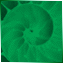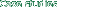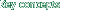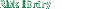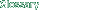PProbability Density Function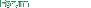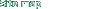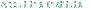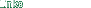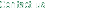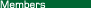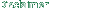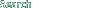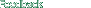### Probability Density Function

 A function that defines the probability distribution of a random variable. By integrating this function between two points, we identify the probability that the random variable will take on a value within the specified interval. See Normal Density Function (diagram).